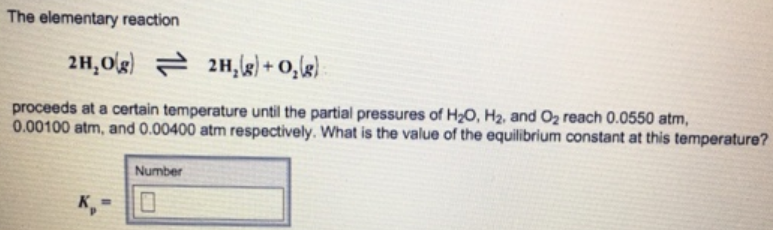# Problem: The elementary reaction 2H2O(g) ⇌ 2H2(g) + O2(g) proceeds at a certain temperature until the partial pressures of H2O, H2, and O2 reach 0.0550 atm, 0.00100 atm, and 0.00400 atm respectively. What is the value of the equilibrium constant at this temperature?

###### FREE Expert Solution###### Problem Details

The elementary reaction

2H2O(g) ⇌ 2H2(g) + O2(g)

proceeds at a certain temperature until the partial pressures of H2O, H2, and O2 reach 0.0550 atm, 0.00100 atm, and 0.00400 atm respectively. What is the value of the equilibrium constant at this temperature?